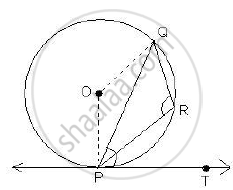# In the figure given below, PQ is a chord of a circle with centre O and PT is a tangent. If ∠QPT = 60°, find ∠PRQ. - Mathematics

In the figure given below, PQ is a chord of a circle with centre O and PT is a tangent. If ∠QPT = 60°, find ∠PRQ.#### Solutionm∠OPT 90º (radius is perpendicular to the tangent)
So, ∠OPQ = ∠OPT - ∠QPT
= 90º - 60º
= 30º
m∠POQ = 2m∠QPT = 2 x 60º = 120º
reflex m∠POQ = 360º - 120º= 240º

/_PQR=1/2 "reflex"/_POQ

=1/2 xx 240^@

=120^@

m/_PRQ=120^@

Concept: Tangent to a Circle
Is there an error in this question or solution?